Courses

# Worksheet - 1: Number System Notes | EduRev

## Class 9 : Worksheet - 1: Number System Notes | EduRev

The document Worksheet - 1: Number System Notes | EduRev is a part of the Class 9 Course Mathematics (Maths) Class 9.
All you need of Class 9 at this link: Class 9

Q.1. Which of the following is an irrational number?
(a)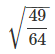(b) √3
(c) 1/2
(d)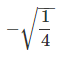Ans.
(b)

Q.2. The number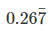in p/q form is
(a) 267/1000
(b) 26/10
(c) 241/900
(d) 241/999
Ans.
(c)
Solution:
let x be the p/q form, x =multiply both side by 100, 100 x =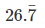...(i)
multiply both side by 10
1000 x =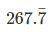....(ii)
1000 x - 100 x =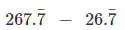= 241
900 x = 241
⇒ x = 241/900
Hence, option (c) is correct

Q.3. Every point on the number line represent, which of the following numbers ?
(a)
Natural numbers
(b)
Irrational number
(c)
Rational number
(d)
Real number
Ans.
(d)

Q.4. Which of the following is not a surd ?
(a)
√6
(b)
√7
(c)
∛343
(d)
√11
Ans.
(c)

Q.5. Show that 3√5 is an irrational number.
Ans.
Let us assume 3√5 is a rational number,
3√5 = p/q, where p and q are co-primes,
√5 = p/3q
Clearly √5 is irrational, while number on right q ≠ 0 are rational
∴ Irrational = Rational
But above deduced can't be right. Therefore our supposition is wrong making 3√5 an irrational number.

Q.6. What is the decimal form of the following no's.
(a) 18/11
(b) 3/26
(c) 1/17
(d) 2/13
Ans.
(a) 18/11 = 1.63636363...
(b) 3/26 = 0.11538461538
(c) 1/17 = 0.05882352941
(d) 2/13 = 0.15384615384

Q.7. The conjugate pair of 2 + √3 is
(a) 2 - √3
(b) 2 + √3
(c) 2√3
(d) 4 - √3
Ans. (a)

Q.8. Simplify: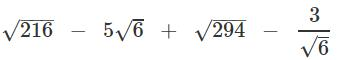Ans.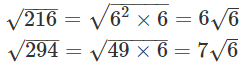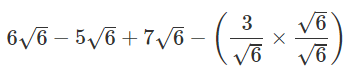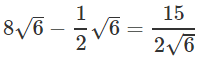Q.9. Rationalise: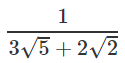Ans.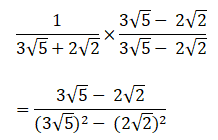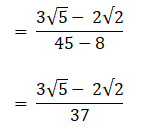Q.10. Find the value of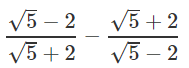Ans.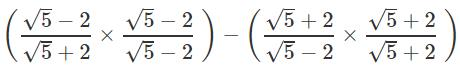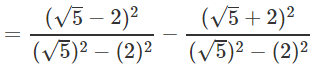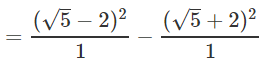= 5+4 - 4√5 - 5 - 4 -  4√5  = -8√5

Q.11. If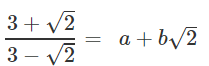find the value of a & b.
Ans.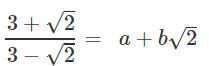Rationalising LHS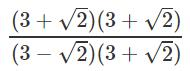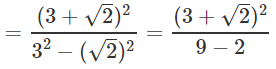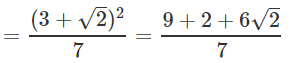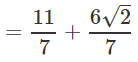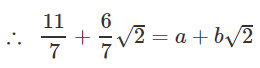∴ a = 11/7 and b = 6/7

Q.12. Evaluate: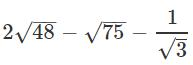Ans.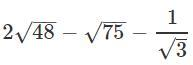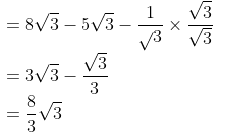Q.13.  If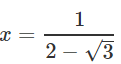,
find the value of x3 - 2x2 - 7x+5
Ans.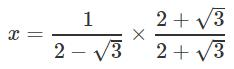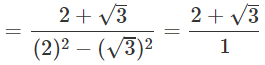x = 2 + √3
x3 - 2x2 - 7x + 5
= x(x- 2x - 7) + 5
= (2 + √3) [(2 + √3)2 - 2(2 + √3) - 7 ]+5
= (2 + √3) [4 + 3+4√3 - 4 + 2√3 - 7]+5
= (2 + √3)(-4 + 2√3) + 5
= (2 + √3) x 2(-2+√3)+5
= 2[(√3 + 2)(√3 - 2)] + 5
= 2[(√3)- 22 ]+5
= 2[3-4]+5
= 2(-1) + 5
= -2+5
= 3

Q.14. Express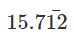in p/q form.
Ans.
let x be the p/q form,
so, x =10x =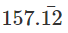1000x =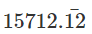1000x - 10x =-990x = 15555
x= 15555/990
= 1037/66

Q.15. Insert five rational no's between 3/5 and 4/5.
Ans.
3/5 and 4/5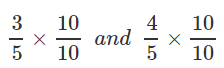30/50 and 40/50
∴ pick any five number between 30 and 40
31/50,  32/50,  36/50,  37/50,  39/50

Q.16. Insert 3 irrational number between 2.6 and 3.8
Ans.
2.6 and 3.8
irrational numbers are non repeating non - terminating
2.61010010001.....
2.802002000200002......
3.604004000400004.......

Q.17. If 27x = 9/3, find x
Ans.
27x = 9/3x
⇒ 33x = (3)2/(3)x
⇒ 33x = 32-x
⇒ 3x = 2-x
⇒ 4x = 2
⇒ x = 4/2
⇒ x = 0.5

Q.18. Write the value of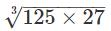Ans.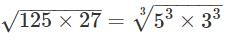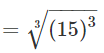= 15

Offer running on EduRev: Apply code STAYHOME200 to get INR 200 off on our premium plan EduRev Infinity!

## Mathematics (Maths) Class 9

47 videos|324 docs|82 tests

,

,

,

,

,

,

,

,

,

,

,

,

,

,

,

,

,

,

,

,

,

;